|

# 逆天了,中凌窗膜的节能效果实在令人惊叹!

在家庭装修中，装贴玻璃窗膜是一种节能环保，健康、经济的方法，但贴对了能给家庭增添一抹舒适，不贴或者贴错了则是给生活增添了一抹烦恼。以下用真实案例来证明贴什么膜才是正确的选择！

小Z是新加坡人士，从事经商活动，在新加坡silvers sea 银海高档公寓新购置一处面积500余平的高档住宅，本以为新的居住环境能为小Z带来更好的生活品质，然而，新家却给小Z带来了不少的烦恼。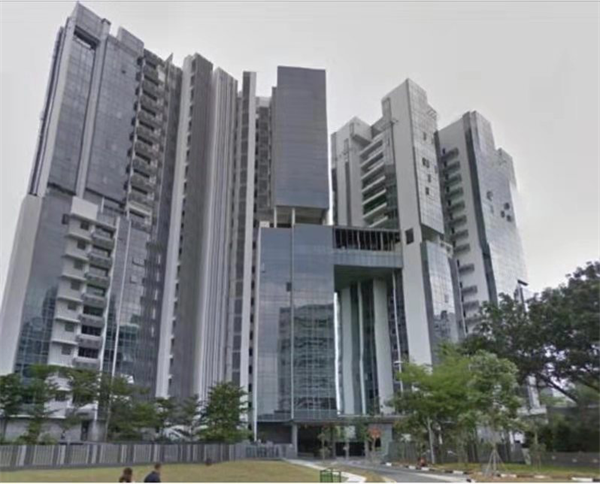（小Z所住新加坡silvers sea银海高档公寓）

小Z居住的楼宇由大面积的玻璃幕墙构成，虽然可以享受更多阳光和美景，但是新加坡常年的高温天气和太阳直晒，导致热量大幅进入室内，小Z为了解决室内灼热问题只能长时间开着空调制冷。Silvers sea是滨海高档公寓，每当小Z想要站到高逼格的透明玻璃窗边，去看看窗外的海边美景时，总会被阳光的灼热和炫目搅得很烦，即使开了空调，窗边和室内的温差还是很大。更恼人的是，每月要为空调付出高额的电费，像去年十月份耗电高达7000余度，这一庞大的数字让小Z怀疑是否是家里空调出了问题，还特意请人来查修空调，结果徒劳无功。

为了尽快解决室内灼热问题，小Z随后就花了1.4万新币（约7万人民币）去购买了3M隔热膜，由于自身对于隔热膜的工艺和产品品质并不了解，只能奔着品牌名气去买。然而，贴完居住一段时间后发现，开始的隔热效果还不错，但时间一长隔热效果就不理想了，从隔热演变成了吸热，使室内温度又不断升高，电费也随之增加。

直到有朋友来家里做客，才了解到导致室内温度高的缘由是没有贴到真正隔热的反射膜。原来之前贴的3M膜是吸热膜，就像海绵一样，能短暂吸收热量，可是吸满了就会产生二次热辐射。小Z这下才恍然大悟，原来是没有贴对膜。

得知小Z的烦恼，她朋友给她推荐了自己在用的中凌窗膜，小Z了解到中凌窗膜是反射热量的原理，通过膜层里的贵金属大幅反射红外热量，从而从根本上解决了隔热问题，使空调运行循环效率增加，不但降低了空调制冷负荷，还能给室内人员带来很高的舒适度，减少空调病的发生。最后小Z便决定撕掉原来的吸热膜、更换中凌的贵金属反射膜。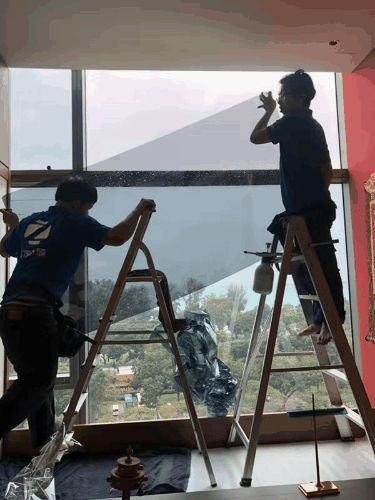（中凌专业施工团队，撕掉3M吸热膜，换上中凌反射膜）

小Z撕掉吸热膜，换上中凌贵金属反射膜后，今年2月耗电骤降至两千多度，比今年1月份少了近一半，二月份家里客人比较多，小Z以为这个月电费应该会很高，后来结算电费时才发现，竟然比12月还低得多，而且12月只住了一周时间。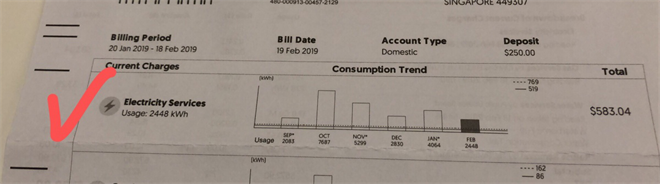（小Z的电费账单，换上中凌膜后，省电超过40%）

经过两个月的实测：电费节约了百分之四十，小Z觉得中国智造的窗膜完全超出了自己的期望值，忍不住晒了朋友圈大加夸赞，”逆天了！真的不敢相信！“，这句话让中凌窗膜的经销商都非常激动。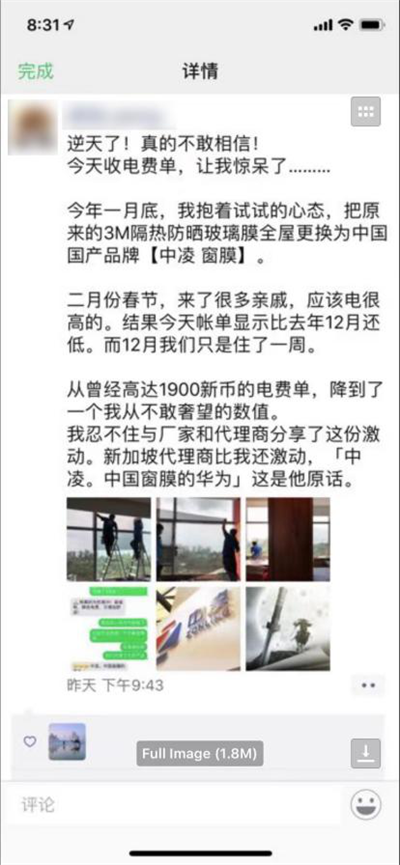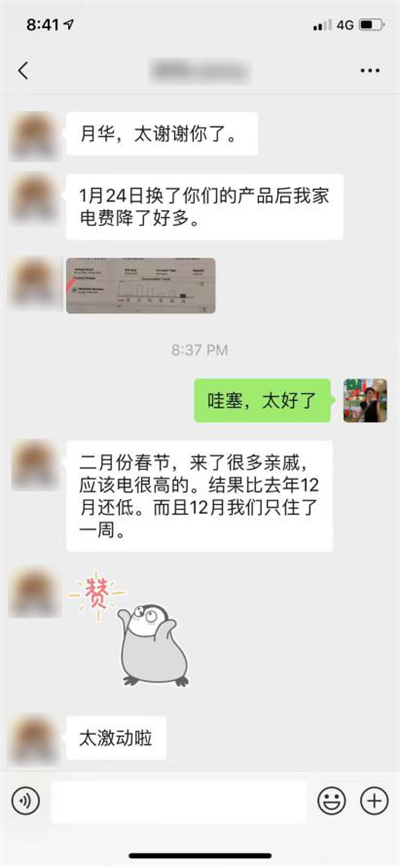（小Z贴中凌膜后的感言，和中凌经销商的对话）

绿色窗膜，节能先锋

真正高隔热的窗膜产品能大幅降低空调能耗，减少碳排放。在上海建科院做的窗膜节能检测中，中凌窗膜的节能效果达到了惊人的24.47%。中凌窗膜作为玻璃上的隐形防护衣，在阳光下首当其冲，不惧烈日骄阳，大幅反射多余的恼人热量，节省了室内的空调能耗，不愧为“节能先锋”的称号。

据了解，中凌窗膜不但有隔热节能的作用，还能阻隔99%紫外线，有效呵护肌肤和眼睛，并且延缓家具的褪色老化，同时还能大幅度提升玻璃的防爆安全性能。

2017年，中凌窗膜获得了新加坡绿色节能建筑产品认证（√√√优秀级别），这是东南亚最权威的绿色建筑产品认证，中凌也是唯一入选的中国窗膜品牌，并且已经在新加坡多个建筑项目上得到运用，比如圣淘沙酒店、樟宜机场等等。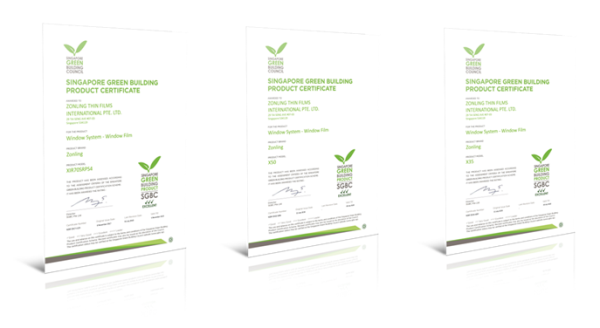（中凌窗膜获得新加坡绿色节能建筑产品认证）

小Z的贴膜故事，让我们看到了中国智造的绿色窗膜，已经走向世界，并且在性能指标上已经超越了一些国际知名品牌。中凌现在已经是国际窗膜协会（IWFA）权威认证的全球9家窗膜制造商之一，而且是生产和技术双认证的企业。我们欣喜地看到，在世界窗膜的版图上已经塑造起了一个中国人自己值得骄傲的绿色窗膜品牌。

“绿色节能环保“的理念已经深入人心，快给家里的玻璃穿上真正隔热的“防晒衣”吧，好窗膜让每一寸阳光都变得更加温馨，享受舒适和惬意的同时还能为绿色节能环保贡献一份力量，何乐而不为呢？

`声明：本文由入驻焦点开放平台的作者撰写，除焦点官方账号外，观点仅代表作者本人，不代表焦点立场错误信息举报电话： 400-099-0099，邮箱：jubao@vip.sohu.com，或点此进行意见反馈，或点此进行举报投诉。`A B C D E F G H J K L M N P Q R S T W X Y Z
A - B - C - D - E
• A
• 鞍山
• 安庆
• 安阳
• 安顺
• 安康
• 澳门
• B
• 北京
• 保定
• 包头
• 巴彦淖尔
• 本溪
• 蚌埠
• 亳州
• 滨州
• 北海
• 百色
• 巴中
• 毕节
• 保山
• 宝鸡
• 白银
• 巴州
• C
• 承德
• 沧州
• 长治
• 赤峰
• 朝阳
• 长春
• 常州
• 滁州
• 池州
• 长沙
• 常德
• 郴州
• 潮州
• 崇左
• 重庆
• 成都
• 楚雄
• 昌都
• 慈溪
• 常熟
• D
• 大同
• 大连
• 丹东
• 大庆
• 东营
• 德州
• 东莞
• 德阳
• 达州
• 大理
• 德宏
• 定西
• 儋州
• 东平
• E
• 鄂尔多斯
• 鄂州
• 恩施
F - G - H - I - J
• F
• 抚顺
• 阜新
• 阜阳
• 福州
• 抚州
• 佛山
• 防城港
• G
• 赣州
• 广州
• 桂林
• 贵港
• 广元
• 广安
• 贵阳
• 固原
• H
• 邯郸
• 衡水
• 呼和浩特
• 呼伦贝尔
• 葫芦岛
• 哈尔滨
• 黑河
• 淮安
• 杭州
• 湖州
• 合肥
• 淮南
• 淮北
• 黄山
• 菏泽
• 鹤壁
• 黄石
• 黄冈
• 衡阳
• 怀化
• 惠州
• 河源
• 贺州
• 河池
• 海口
• 红河
• 汉中
• 海东
• 怀来
• I
• J
• 晋中
• 锦州
• 吉林
• 鸡西
• 佳木斯
• 嘉兴
• 金华
• 景德镇
• 九江
• 吉安
• 济南
• 济宁
• 焦作
• 荆门
• 荆州
• 江门
• 揭阳
• 金昌
• 酒泉
• 嘉峪关
K - L - M - N - P
• K
• 开封
• 昆明
• 昆山
• L
• 廊坊
• 临汾
• 辽阳
• 连云港
• 丽水
• 六安
• 龙岩
• 莱芜
• 临沂
• 聊城
• 洛阳
• 漯河
• 娄底
• 柳州
• 来宾
• 泸州
• 乐山
• 六盘水
• 丽江
• 临沧
• 拉萨
• 林芝
• 兰州
• 陇南
• M
• 牡丹江
• 马鞍山
• 茂名
• 梅州
• 绵阳
• 眉山
• N
• 南京
• 南通
• 宁波
• 南平
• 宁德
• 南昌
• 南阳
• 南宁
• 内江
• 南充
• P
• 盘锦
• 莆田
• 平顶山
• 濮阳
• 攀枝花
• 普洱
• 平凉
Q - R - S - T - W
• Q
• 秦皇岛
• 齐齐哈尔
• 衢州
• 泉州
• 青岛
• 清远
• 钦州
• 黔南
• 曲靖
• 庆阳
• R
• 日照
• 日喀则
• S
• 石家庄
• 沈阳
• 双鸭山
• 绥化
• 上海
• 苏州
• 宿迁
• 绍兴
• 宿州
• 三明
• 上饶
• 三门峡
• 商丘
• 十堰
• 随州
• 邵阳
• 韶关
• 深圳
• 汕头
• 汕尾
• 三亚
• 三沙
• 遂宁
• 山南
• 商洛
• 石嘴山
• T
• 天津
• 唐山
• 太原
• 通辽
• 铁岭
• 泰州
• 台州
• 铜陵
• 泰安
• 铜仁
• 铜川
• 天水
• 天门
• W
• 乌海
• 乌兰察布
• 无锡
• 温州
• 芜湖
• 潍坊
• 威海
• 武汉
• 梧州
• 渭南
• 武威
• 吴忠
• 乌鲁木齐
X - Y - Z
• X
• 邢台
• 徐州
• 宣城
• 厦门
• 新乡
• 许昌
• 信阳
• 襄阳
• 孝感
• 咸宁
• 湘潭
• 湘西
• 西双版纳
• 西安
• 咸阳
• 西宁
• 仙桃
• 西昌
• Y
• 运城
• 营口
• 盐城
• 扬州
• 鹰潭
• 宜春
• 烟台
• 宜昌
• 岳阳
• 益阳
• 永州
• 阳江
• 云浮
• 玉林
• 宜宾
• 雅安
• 玉溪
• 延安
• 榆林
• 银川
• Z
• 张家口
• 镇江
• 舟山
• 漳州
• 淄博
• 枣庄
• 郑州
• 周口
• 驻马店
• 株洲
• 张家界
• 珠海
• 湛江
• 肇庆
• 中山
• 自贡
• 资阳
• 遵义
• 昭通
• 张掖
• 中卫

1室1厅1厨1卫1阳台

1
2
3
4
5

0
1
2

1

1

0
1
2
3报名成功，资料已提交审核A B C D E F G H J K L M N P Q R S T W X Y Z
A - B - C - D - E
• A
• 鞍山
• 安庆
• 安阳
• 安顺
• 安康
• 澳门
• B
• 北京
• 保定
• 包头
• 巴彦淖尔
• 本溪
• 蚌埠
• 亳州
• 滨州
• 北海
• 百色
• 巴中
• 毕节
• 保山
• 宝鸡
• 白银
• 巴州
• C
• 承德
• 沧州
• 长治
• 赤峰
• 朝阳
• 长春
• 常州
• 滁州
• 池州
• 长沙
• 常德
• 郴州
• 潮州
• 崇左
• 重庆
• 成都
• 楚雄
• 昌都
• 慈溪
• 常熟
• D
• 大同
• 大连
• 丹东
• 大庆
• 东营
• 德州
• 东莞
• 德阳
• 达州
• 大理
• 德宏
• 定西
• 儋州
• 东平
• E
• 鄂尔多斯
• 鄂州
• 恩施
F - G - H - I - J
• F
• 抚顺
• 阜新
• 阜阳
• 福州
• 抚州
• 佛山
• 防城港
• G
• 赣州
• 广州
• 桂林
• 贵港
• 广元
• 广安
• 贵阳
• 固原
• H
• 邯郸
• 衡水
• 呼和浩特
• 呼伦贝尔
• 葫芦岛
• 哈尔滨
• 黑河
• 淮安
• 杭州
• 湖州
• 合肥
• 淮南
• 淮北
• 黄山
• 菏泽
• 鹤壁
• 黄石
• 黄冈
• 衡阳
• 怀化
• 惠州
• 河源
• 贺州
• 河池
• 海口
• 红河
• 汉中
• 海东
• 怀来
• I
• J
• 晋中
• 锦州
• 吉林
• 鸡西
• 佳木斯
• 嘉兴
• 金华
• 景德镇
• 九江
• 吉安
• 济南
• 济宁
• 焦作
• 荆门
• 荆州
• 江门
• 揭阳
• 金昌
• 酒泉
• 嘉峪关
K - L - M - N - P
• K
• 开封
• 昆明
• 昆山
• L
• 廊坊
• 临汾
• 辽阳
• 连云港
• 丽水
• 六安
• 龙岩
• 莱芜
• 临沂
• 聊城
• 洛阳
• 漯河
• 娄底
• 柳州
• 来宾
• 泸州
• 乐山
• 六盘水
• 丽江
• 临沧
• 拉萨
• 林芝
• 兰州
• 陇南
• M
• 牡丹江
• 马鞍山
• 茂名
• 梅州
• 绵阳
• 眉山
• N
• 南京
• 南通
• 宁波
• 南平
• 宁德
• 南昌
• 南阳
• 南宁
• 内江
• 南充
• P
• 盘锦
• 莆田
• 平顶山
• 濮阳
• 攀枝花
• 普洱
• 平凉
Q - R - S - T - W
• Q
• 秦皇岛
• 齐齐哈尔
• 衢州
• 泉州
• 青岛
• 清远
• 钦州
• 黔南
• 曲靖
• 庆阳
• R
• 日照
• 日喀则
• S
• 石家庄
• 沈阳
• 双鸭山
• 绥化
• 上海
• 苏州
• 宿迁
• 绍兴
• 宿州
• 三明
• 上饶
• 三门峡
• 商丘
• 十堰
• 随州
• 邵阳
• 韶关
• 深圳
• 汕头
• 汕尾
• 三亚
• 三沙
• 遂宁
• 山南
• 商洛
• 石嘴山
• T
• 天津
• 唐山
• 太原
• 通辽
• 铁岭
• 泰州
• 台州
• 铜陵
• 泰安
• 铜仁
• 铜川
• 天水
• 天门
• W
• 乌海
• 乌兰察布
• 无锡
• 温州
• 芜湖
• 潍坊
• 威海
• 武汉
• 梧州
• 渭南
• 武威
• 吴忠
• 乌鲁木齐
X - Y - Z
• X
• 邢台
• 徐州
• 宣城
• 厦门
• 新乡
• 许昌
• 信阳
• 襄阳
• 孝感
• 咸宁
• 湘潭
• 湘西
• 西双版纳
• 西安
• 咸阳
• 西宁
• 仙桃
• 西昌
• Y
• 运城
• 营口
• 盐城
• 扬州
• 鹰潭
• 宜春
• 烟台
• 宜昌
• 岳阳
• 益阳
• 永州
• 阳江
• 云浮
• 玉林
• 宜宾
• 雅安
• 玉溪
• 延安
• 榆林
• 银川
• Z
• 张家口
• 镇江
• 舟山
• 漳州
• 淄博
• 枣庄
• 郑州
• 周口
• 驻马店
• 株洲
• 张家界
• 珠海
• 湛江
• 肇庆
• 中山
• 自贡
• 资阳
• 遵义
• 昭通
• 张掖
• 中卫• 手机• 分享
• 设计
免费设计
• 计算器
装修计算器
• 入驻
合作入驻
• 联系
联系我们
• 置顶
返回顶部